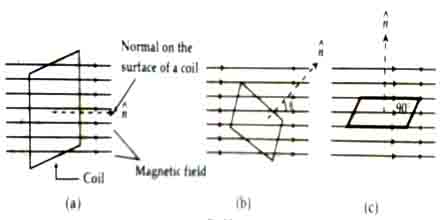# Surface Vector

The direction represented by the normal drawn on a plane or surface is the vector of that surface. In this case the plane is the surface. The normal drawn on a surface is A called the surface vector of that surface.. In the following figure surface vector (ň) has been shown by dotted line. Here the coil is the surface.It x is the uniform continuous scalar function in a field, then a surface may be considered passing through a point in that field in which the value of that function at each point becomes equal to the value of that point. Uniform potential surface is the best example of this type of function.

If the position function at a point is v, and co-ordinate of that point in rectangular co-ordinate system is (x, y, z), then (δv/δx) is the rate of change of v with respect to A at that point along Y-axis.